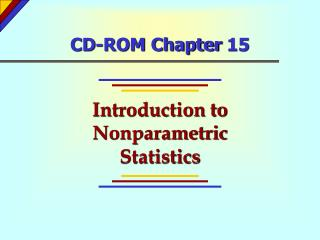DownloadDownload PresentationCD-ROM Chapter 15

# CD-ROM Chapter 15

Download Presentation## CD-ROM Chapter 15

- - - - - - - - - - - - - - - - - - - - - - - - - - - E N D - - - - - - - - - - - - - - - - - - - - - - - - - - -
##### Presentation Transcript

1. CD-ROM Chapter 15 Introduction to Nonparametric Statistics

2. Chapter 15 - Chapter Outcomes After studying the material in this chapter, you should be able to: Recognize when and how to use the runs test and testing for randomness. Know when and how to perform a Mann-Whitney U test. Recognize the situations for which the Wilcoxon signed rank test applies and be able to use it in a decision-making context. Perform nonparametric analysis of variance using the Kruskal-Wallis one-way ANOVA.

3. Nonparametric Statistics Nonparametric statistical procedures are those statistical methods that do not concern themselves with population distributions and/or parameters.

4. The Runs Test The runs test is a statistical procedure used to determine whether the pattern of occurrences of two types of observations is determined by a random process.

5. The Runs Test A run is a succession of occurrences of a certain type preceded and followed by occurrences of the alternate type or by no occurrences at all.

6. The Runs Test(Table 15-1)

7. The Runs Test(Small Sample Example) H0: Computer-generated numbers are random between 0.0 and 1.0. HA: Computer-generated numbers are not random . --- + - ++ -- ++ --- ++ -- ++ Runs: 1 2 3 4 5 6 7 8 9 10 There are r = 10 runs From runs table (Appendix K) with n1 = 9 and n2 = 11, the critical value of r is 6

8. The Runs Test(Small Sample Example) Test Statistic: r = 10 runs Critical Values from Runs Table: Possible Runs: 1 2 3 4 5 6 7 8 9 10 11 12 13 14 15 16 17 18 19 20 Reject H0 Reject H0 Do not reject H0 Decision: Since r = 10, we do not reject the null hypothesis.

9. Large Sample Runs Test MEAN AND STANDARD DEVIATION FOR r where: n1 = Number of occurrences of first type n2 = Number of occurrences of second type

10. Large Sample Runs Test TEST STATISTIC FOR LARGE SAMPLE RUNS TEST

11. Large Sample Runs Test(Example 15-2) Table 15-2 OOOUOOUOUUOOUUOOOOUUOUUOOO UUUOOOOUUOOUUUOUUOOUUUUU OOOUOUUOOOUOOOOUUUOUUOOOU OOUUOUOOUUUOUUOOOOUUUOOO n1 = 53 “O’s” n2 = 47 “U’s” r = 45 runs

12. Large Sample Runs Test (Example 15-2) H0: Yogurt fill amounts are randomly distributed above and below 24-ounce level. H1: Yogurt fill amounts are not randomly distributed above and below 24-ounce level.  = 0.05 Rejection Region  /2 = 0.025 Rejection Region  /2 = 0.025 Since z= -1.174 > -1.96 and < 1.96, we do not reject H0,

13. Mann-Whitney U Test The Mann Whitney U test can be used to compare two samples from two populations if the following assumptions are satisfied: • The two samples are independent and random. • The value measured is a continuous variable. • The measurement scale used is at least ordinal. • If they differ, the distributions of the two populations will differ only with respect to the central location.

14. Mann-Whitney U Test U-STATISTICS where: n1 and n2 are the two sample sizes R1 and R2 = Sum of ranks for samples 1 and 2

15. Mann-Whitney U Test- Large Samples - MEAN AND STANDARD DEVIATION FOR THE U-STATISTIC where: n1 and n2 = Sample sizes from populations 1 and 2

16. Mann-Whitney U Test- Large Samples - MANN-WHITNEY U-TEST STATISTIC

17. Mann-Whitney U Test(Example 15-4) Rejection Region  = 0.05 Since z= -1.027 > -1.645, we do not reject H0,

18. Wilcoxon Matched-Pairs Test The Wilcoxon matched pairs signed rank test can be used in those cases where the following assumptions are satisfied: • The differences are measured on a continuous variable. • The measurement scale used is at least interval. • The distribution of the population differences is symmetric about their median.

19. Wilcoxon Matched-Pairs Test WILCOXON MEAN AND STANDARD DEVIATION where: n = Number of paired values

20. Wilcoxon Matched-Pairs Test WILCOXON TEST STATISTIC

21. Kruskal-Wallis One-Way Analysis of Variance Kruskal-Wallis one-way analysis of variance can be used in one-way analysis of variance if the variables satisfy the following: • They have a continuous distribution. • The data are at least ordinal. • The samples are independent. • The samples come from populations whose only possible difference is that at least one may have a different central location than the others.

22. Kruskal-Wallis One-Way Analysis of Variance H-STATISTIC where: N = Sum of sample sizes in all samples k = Number of samples Ri = Sum of ranks in the ith sample ni = Size of the ith sample

23. Kruskal-Wallis One-Way Analysis of Variance CORRECTION FOR TIED RANKINGS where: g = Number of different groups of ties ti = Number of tied observations in the ith tied group of scores N = Total number of observations

24. Kruskal-Wallis One-Way Analysis of Variance H-STATISTIC CORRECTED FOR TIED RANKINGS

25. • Kruskal-Wallis One-Way Analysis of Variance • Mann-Whitney U Test • Nonparametric Statistical Procedure Key Terms • Run • Runs Test • Wilcoxon Test